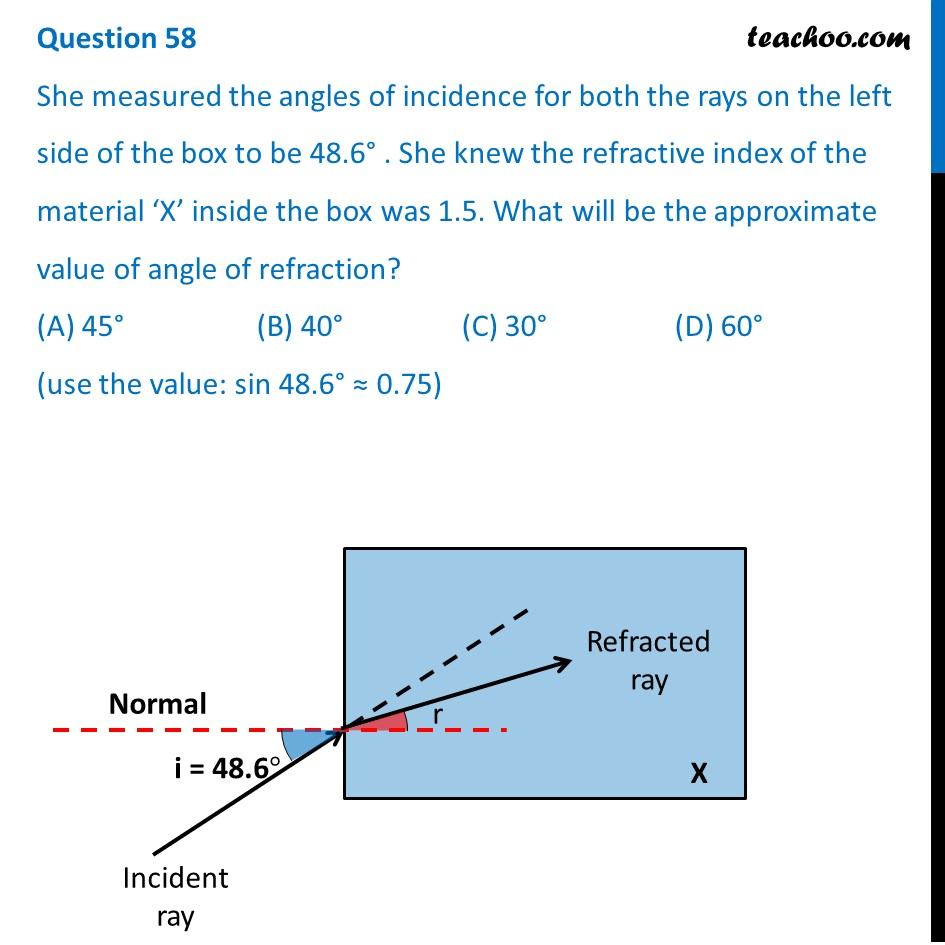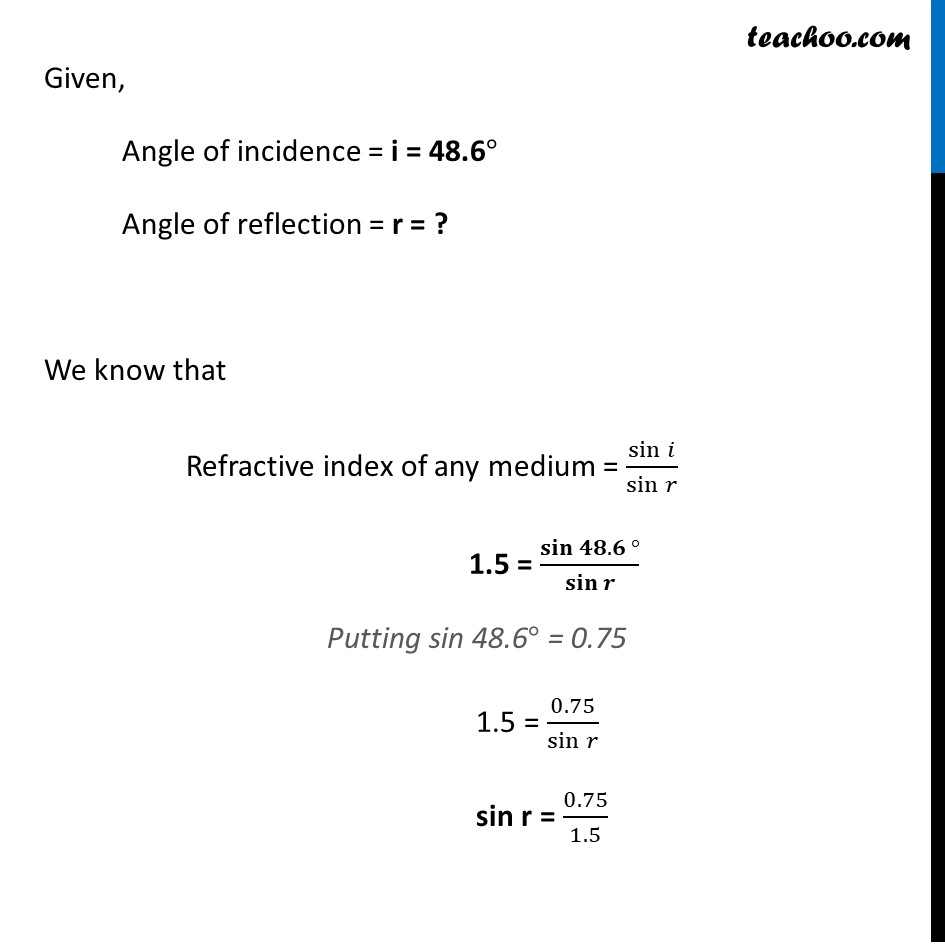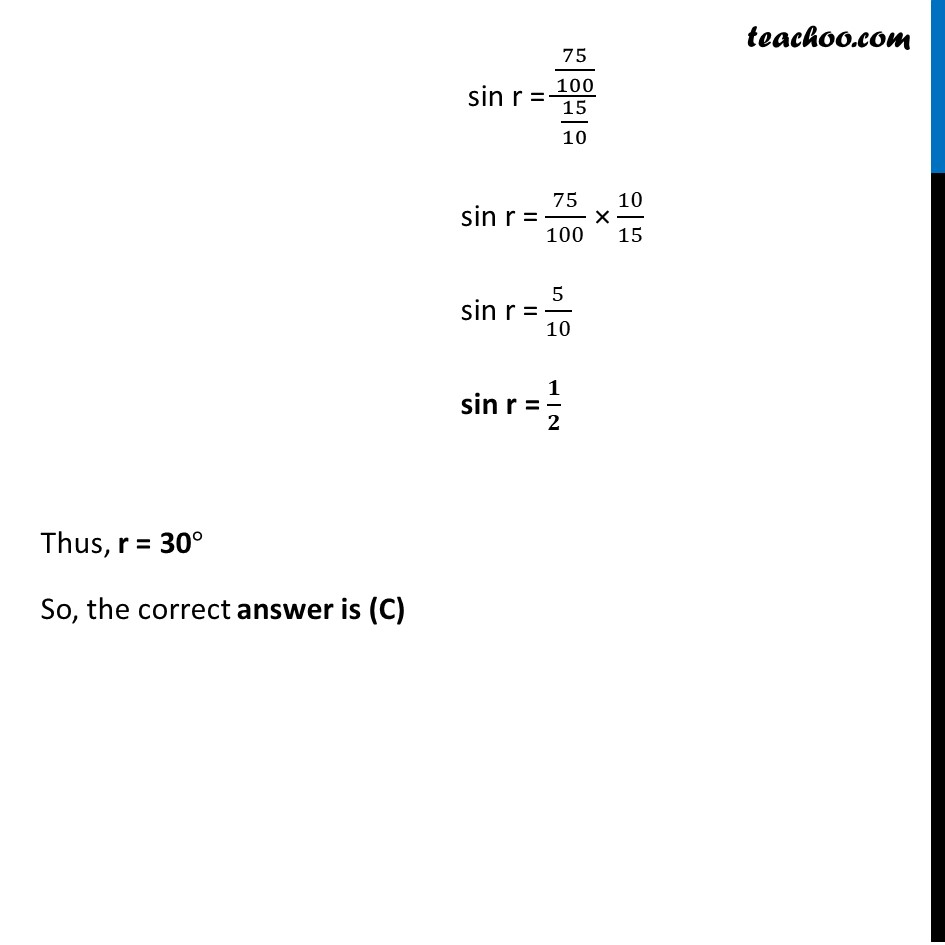Solutions - CBSE Class 10 Sample Paper for 2022 Boards - Science [MCQ]

Class 10
Solutions to CBSE Sample Paper - Science Class 10

## (D) 60 ° (use the value: sin 48.6 ° ≈ 0.75)Learn in your speed, with individual attention - Teachoo Maths 1-on-1 Class

### Transcript

Question 58 She measured the angles of incidence for both the rays on the left side of the box to be 48.6° . She knew the refractive index of the material ‘X’ inside the box was 1.5. What will be the approximate value of angle of refraction? (A) 45° (B) 40° (C) 30° (D) 60° (use the value: sin 48.6° ≈ 0.75) Given, Angle of incidence = i = 48.60 Angle of reflection = r = ? We know that Refractive index of any medium = sin⁡𝑖/sin⁡𝑟 1.5 = 𝐬𝐢𝐧⁡〖𝟒𝟖.𝟔〗/𝐬𝐢𝐧⁡𝒓 Putting sin 48.6° = 0.75 1.5 = 0.75/sin⁡𝑟 sin r = 0.75/1.5 sin r = (75/100)/(15/10) sin r = 75/100 × 10/15 sin r = 5/10 sin r = 𝟏/𝟐 Thus, r = 300 So, the correct answer is (C)